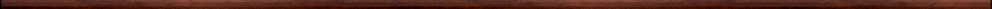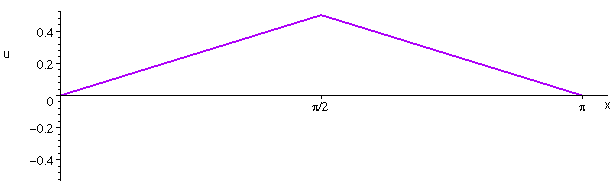Maple worksheets on partial differential equations and the 1-dimensional wave equationDifferential equations:

They are all compatible with Classic Worksheet Maple 10.Introduction to partial differential equations - PDEintro.mws

• Introductory examples

The wave equation - wave_eq1.mws

• Derivation of the 1-dimensional wave equation
• A solution of the 1-dimensional wave equation
• Standing waves

The wave equation - wave_eq2.mws

• A special case for the solution of the wave equation
• Examples of standing wave solutions for the wave equation
• D'Alembert's travelling wave solution for the wave equation
• An example of d'Alembert's solution for the wave equation
• Historical note

Finding solutions to partial differential equations by separating the variables - PDEsepvar.mws

• Examples

A procedure for constructing solutions to the wave equation - waveproc.mws

• A procedure for constructing solutions to the wave equation:  convert(.., wave,x)
• Examples

Procedures for Fourier series and Fourier transforms - fourier.zip

Top of page

Main index